The topic of color replacement in images has interested me long before I started programming. Playing around with free tools and simple processing approaches (e.g. hue replacement in the HSV space) never felt “satisfying” in relation to what I was imagining when specifying the replacement colors - there’s always some fidgety part such as specifying thresholds which causes sharp edges or other strange-looking artifacts, or simply the replacement hues seem “off”. Various papers exist that do seem to do a good job (see this and this for some examples) and Photoshop naturally has an implementation, but when the time came to choose a topic for my final project in numerical optimization, I thought it was a good opportunity to take a shot at the problem myself. It’s a challenging problem and while the results are far from perfect, I’m pretty happy with how it turned out.

### Some results

Original image: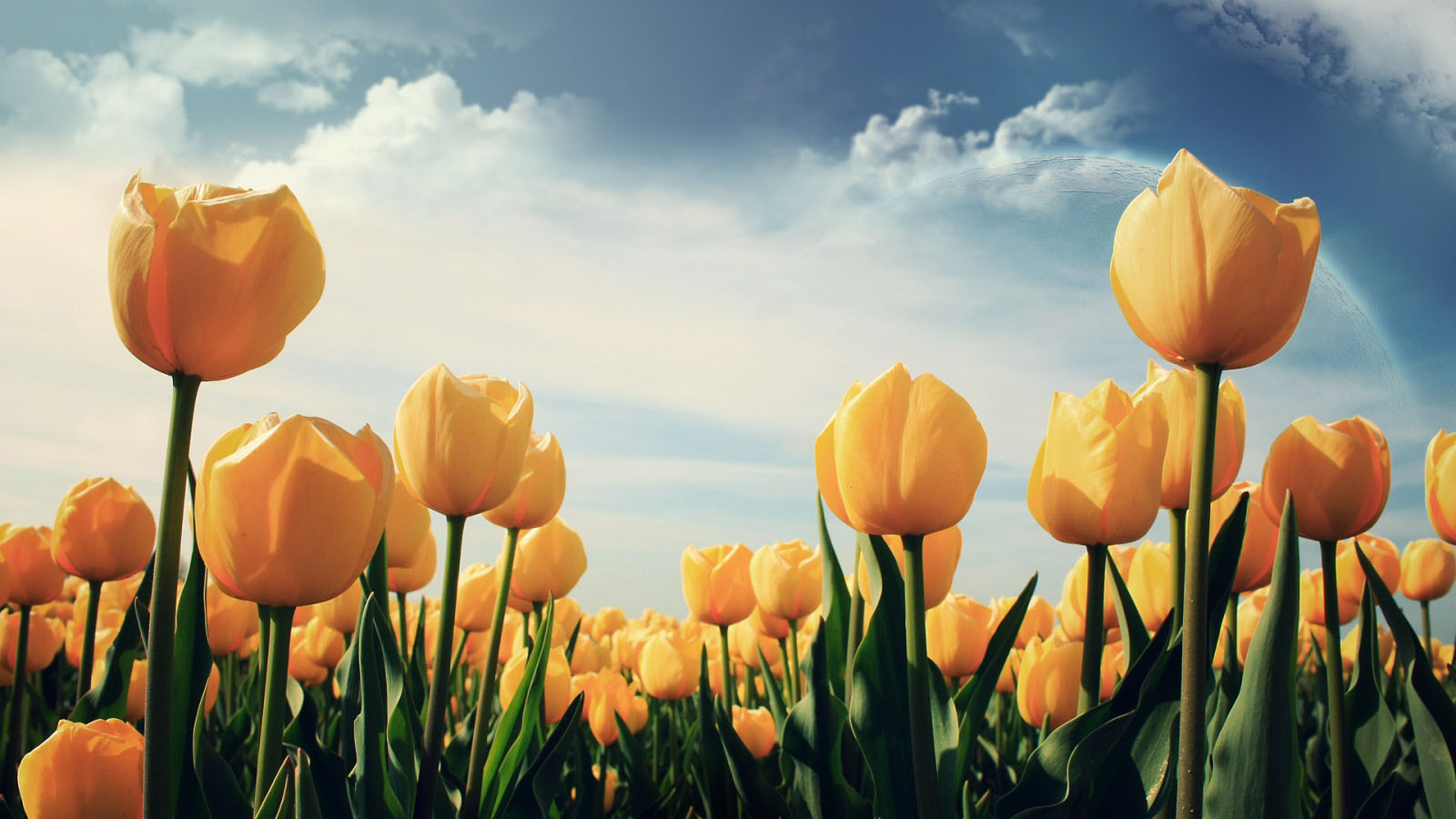Flowers replaced to red: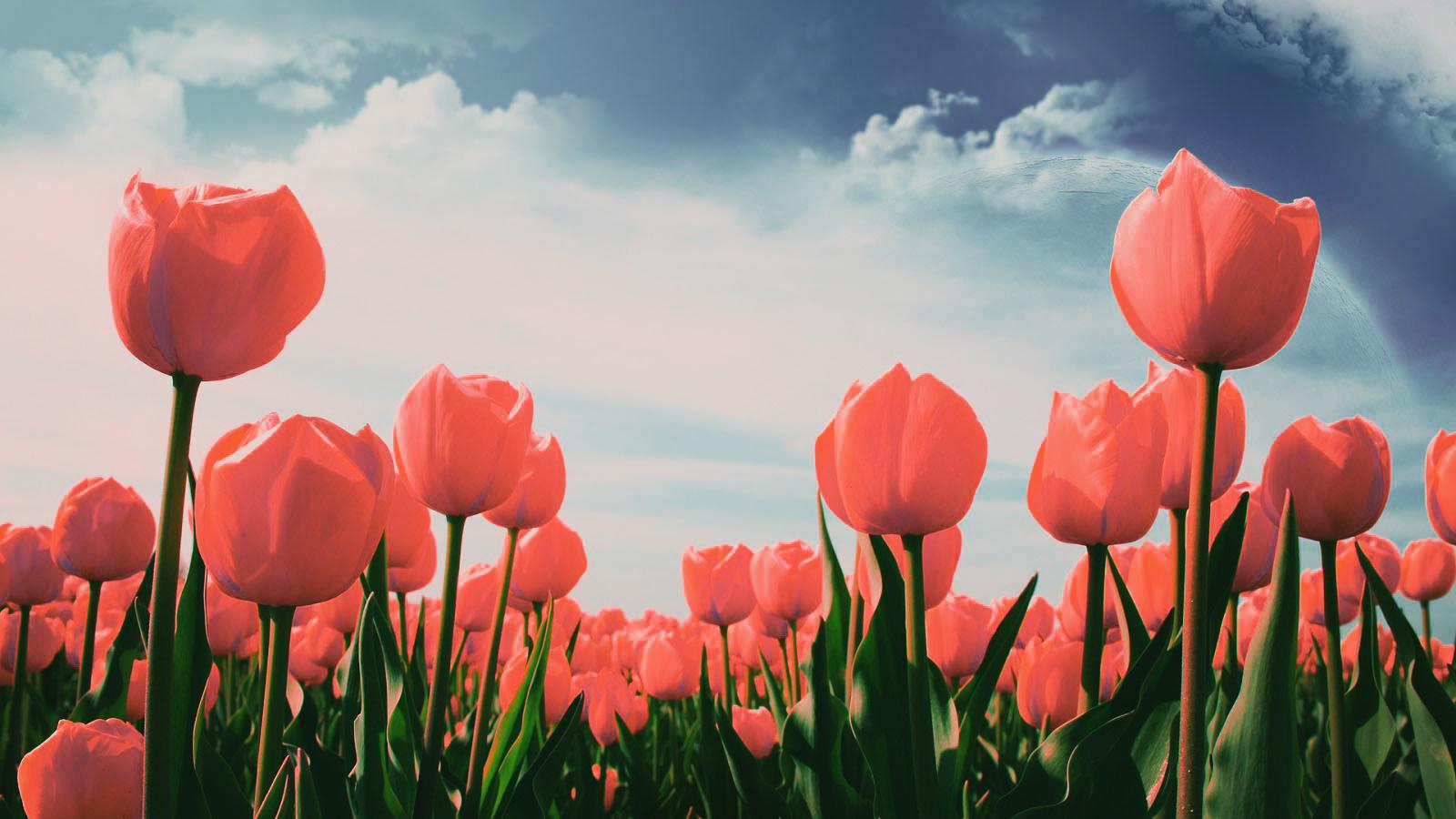Stems replaced to pink: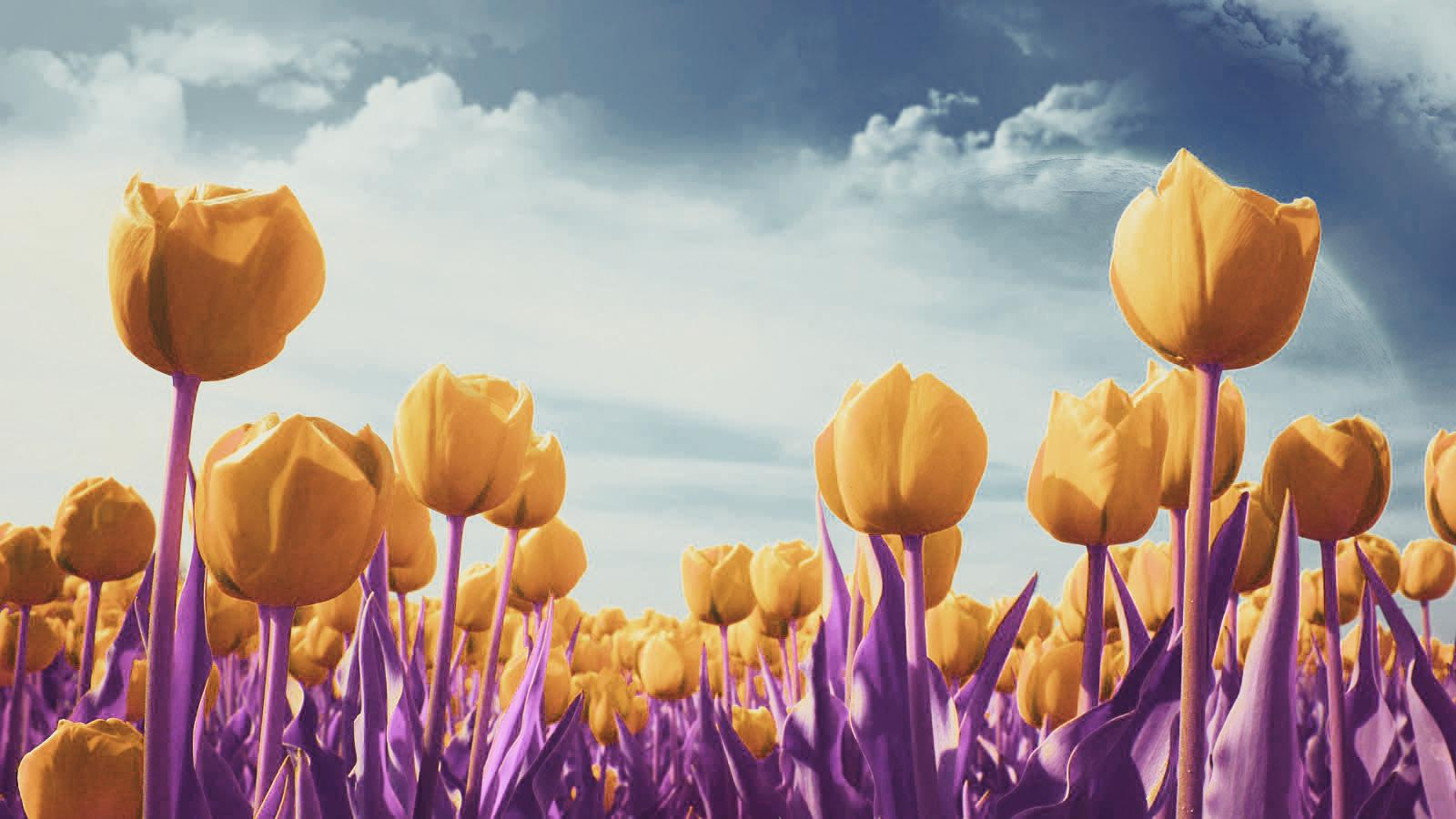### The approach

The general idea was to have a user specify an image, a list of colors to be replaced, a list of colors to replace them with, and a list of colors to stay the same. We would then perform some optimization process using all that and output the new image, with all the requirements met (and also hopefully looking “nice” and without strange artifacts).

After a few false starts, I arrived at the following formulation:

#### Inputs / constants

$$I \in \mathbb{R}^{n \times 3}$$ - Flattened image

$$C_1 \in \mathbb{R}^{m \times 3}$$ - Colors to replace (including fixed colors)

$$C_2 \in \mathbb{R}^{m \times 3}$$ - Colors to replace with (including fixed colors)

#### Variables

$T \in \mathbb{R}^{3 \times k}$ $B \in \mathbb{R}^{k \times 3}$ $N \in \mathbb{R}^{k \times 3}$

#### Desired transformation: $$\sigma(\sigma(IT)N)$$

This will yield the (flattened) image with colors replaced. ($$\sigma$$ refers to the Sigmoid function.)

#### Problem

Minimize:

$\sum|B| + \sum|N| + \sum|\sigma(IT)|$

Subject to:

$\sigma(\sigma(IT)B) = I$ $\sigma(\sigma(C_1T)N) = C_2$

The intuition is to transform the image to some “latent color space” (with $$k$$ components) using a first nonlinear transformation and then convert from that space back to RGB while replacing the colors according to the requirements (which also includes a list of “fixed” colors). The two constraints enforce the requirements while the objective aims to arrive at a “sparse” intermediate representation for regularity and smoothness.

The variable $$B$$ appearing in the objective and the first constraint isn’t directly used in the final creation of the target image. However, I’ve found that it improves the results; my hand-wavy explanation for that (and original reason for including it) is that it forces the transformation to intermediate representation to be a meaningful representation of the entire original image, not just the color requirements.

In practice this problem is hard to optimize, so I moved the constraints to the objective:

#### Minimize “loss” function

$J(\theta) = \frac{1}{...}\sum|B| + \frac{1}{...}\sum|N| + \frac{1}{...}\sum|\sigma(IT)| +$ $+ \lambda[ \frac{1}{I_{rows}}\sum_{i=1}^{I_{rows}}{||(I - \sigma(\sigma(IT)B))_{i}||_2} + \frac{1}{C_{2;rows}}\sum_{i=1}^{C_{2;rows}}{||(C_2 - \sigma(\sigma(C_1T)N))_i||_2}]$

Using $$\lambda$$ as a parameter for weighting the objective vs. the constraint violation penalty.

This is then optimized using BFGS and a light touch of the penalty method.

### Intermediate representations

We can look at some of the intermediate representation channels to get an idea of what the transformation is doing: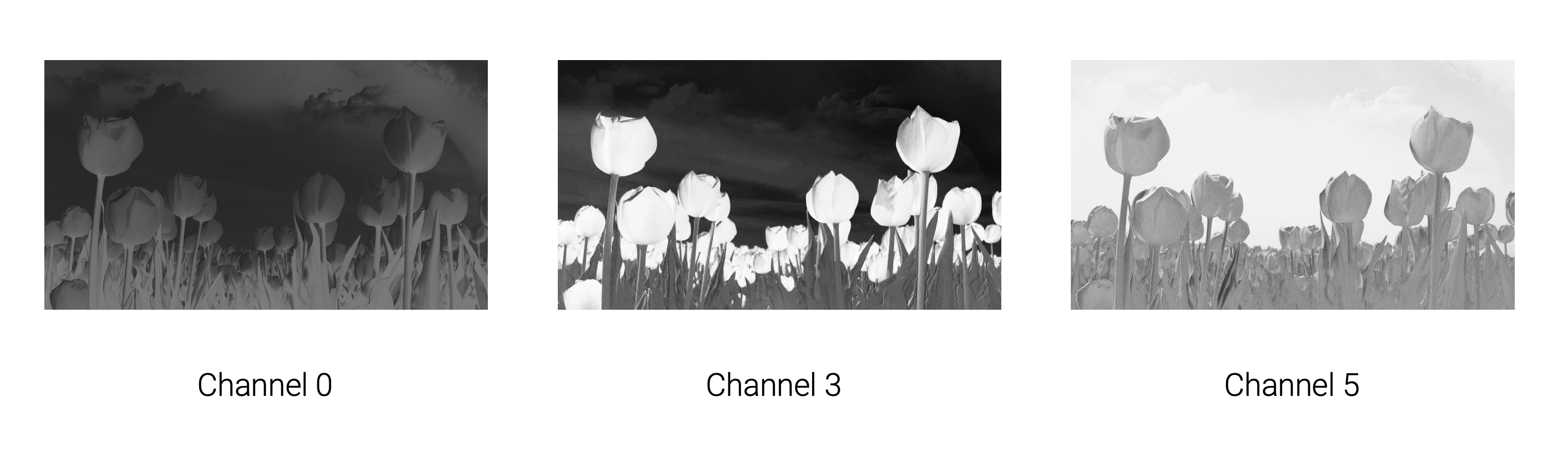### More examples

Other examples include changing a purple ice cream scoop to red: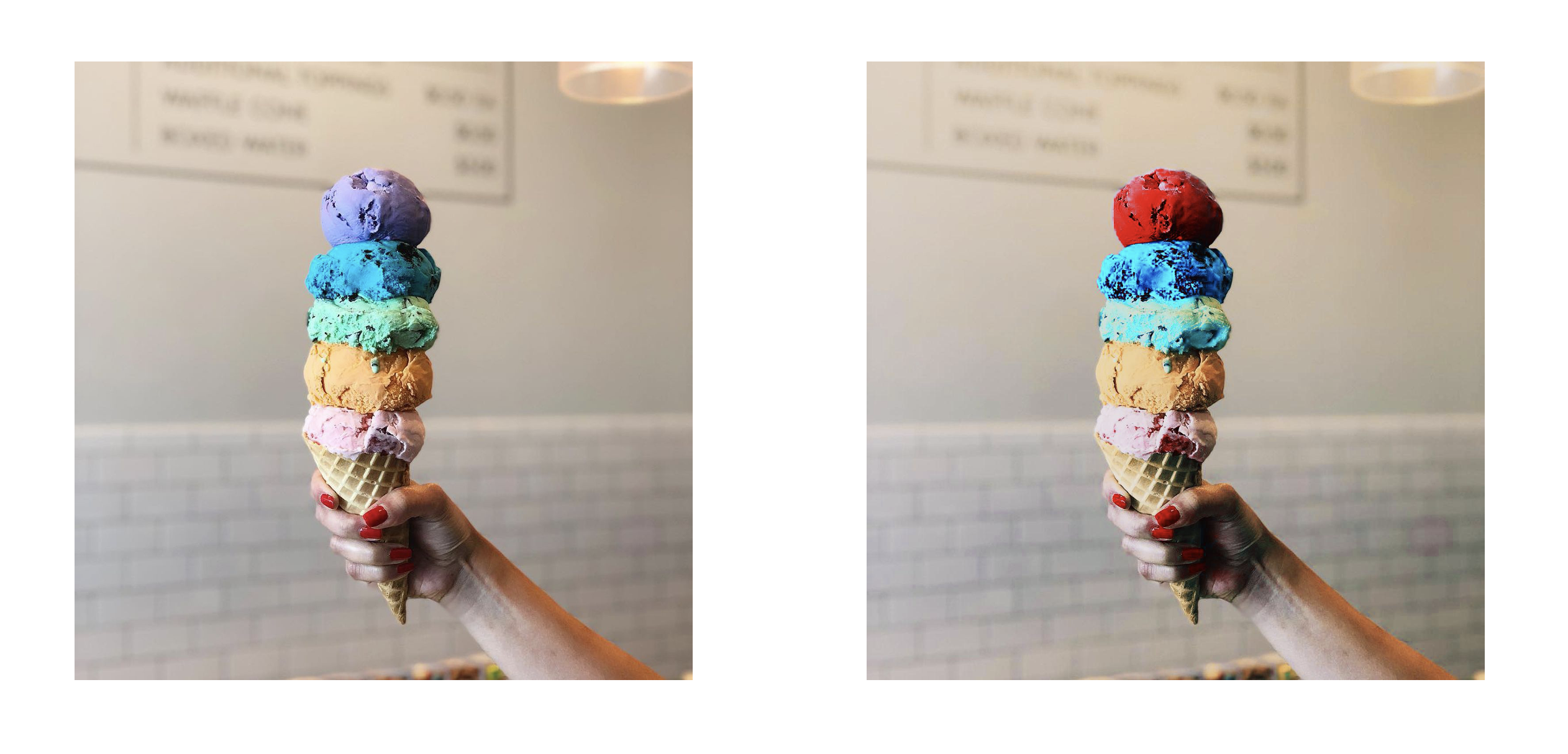And changing the color of my shirt from blue to red:(Yeah, I like red.)

### Code & more

The code can be found here, along with my presentation slides. These include some of the gradient derivation.